# Entering Times Quickly in Microsoft Excel 2010

To enter the times quickly, we use the Timevalue, If, Left, and Right functions in Microsoft Excel 2010.

IF: - Check whether a condition is met and returns one value if True and another value if False.

The syntax of “IF” function =if(logical test,[value_if_true],[value_if_false])

Firstly, the formula will do the logical test, what if the output of logical test is true and false.

For example: Cell A2 and A3 contain the number 3 and 5. If the number is 3, the formula should display “Yes”, else “No”.

=IF (A1=3,"Yes","No")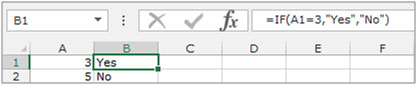TIMEVALUE: - To convert a text time to Excel serial number for a particular time, a number from 0 (12:00:00 Am) to 0.999988426 (11:59:59 PM), format the number with a time format after entering the formula.

The syntax of TIMEVALUE formula:-  =TIMEVALUE(time_text)

For example: - Cell A1 contains the time as text, and we want to convert it into time value.

• Write the formula in cell B1
• =TIMEVALUE(A1)
• Press enter on your keyboard
• The function will convert the text into time format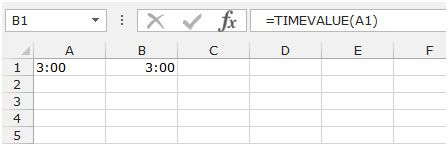LEFT: Returns the first character(s) in a text string based on the number of characters specified.

Syntax of “LEFT” function:            =LEFT (text,[num_chars])

Example: Cell A1contains the text “Broncho Billy Anderson”

=LEFT (A1, 7), function will return “Broncho”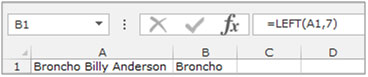RIGHT: Returns the number of characters as specified starting from the right-most character in the string.

Syntax of “RIGHT” function:        =RIGHT (text, [num_chars])

Example: Cell A1contains the text “Broncho Billy Anderson”

=RIGHT (A1, 8), function will return “Anderson”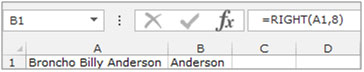Let’s take an example to understand how we can enter the time quickly, without having to use a colon to separate hours and minutes.

We have number list in column A. To convert the numbers into times, follow below given steps:-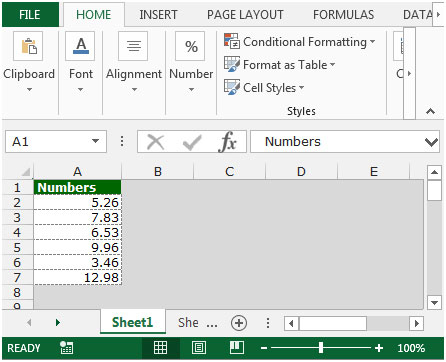• Write the formula in cell B2.
• =TIMEVALUE(IF(A2<1000, LEFT(A2,1),LEFT(A2,2))&":"&RIGHT(A2,2))
• Press Enter on your keyboard.
• Copy the same formula and paste in the range B2:B7.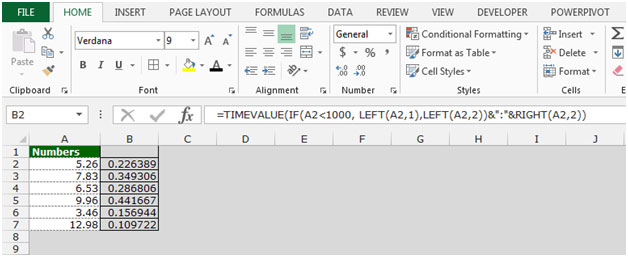• The formula gives the output in general format.

Now follow below given steps to change the range format in to HH:MM

• Press Ctrl+1, Format cells dialog box will appear.
• In the number tab, select Custom.
• In the type box, write the format “hh:mm” and click on OK.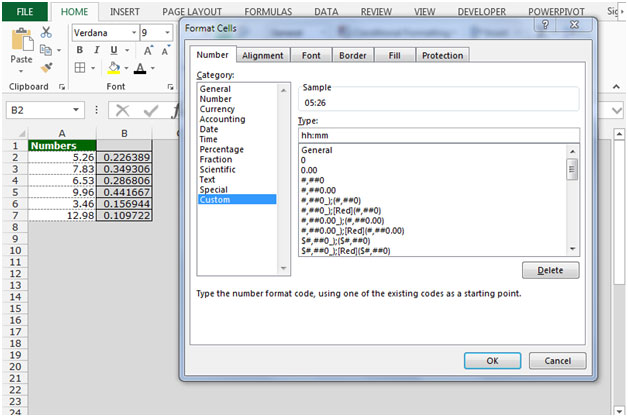• The formula will convert each numerical value in column A to a properly formatted time value.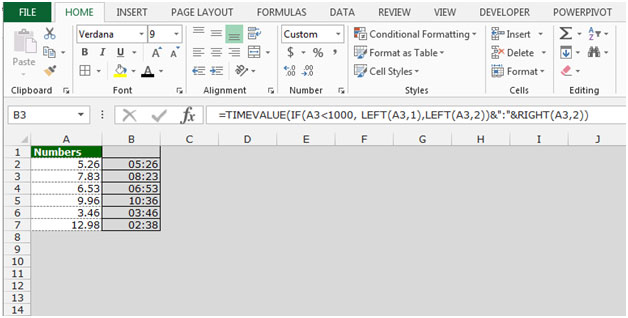This is the way we can add the text values representing time in Microsoft Excel.

Terms and Conditions of use

The applications/code on this site are distributed as is and without warranties or liability. In no event shall the owner of the copyrights, or the authors of the applications/code be liable for any loss of profit, any problems or any damage resulting from the use or evaluation of the applications/code.